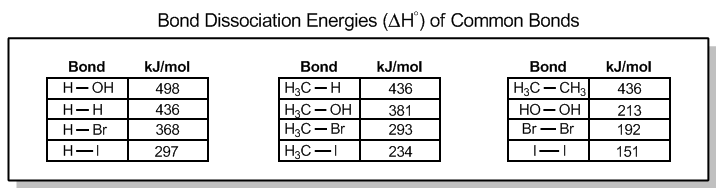Start typing, then use the up and down arrows to select an option from the list.# Organic Chemistry

Learn the toughest concepts covered in Organic Chemistry with step-by-step video tutorials and practice problems by world-class tutors.

6. Thermodynamics and Kinetics

Bond dissociation energies describe the strength of chemical bonds. They can be determined experimentally. With that said, we’ll need some basic math for this section to determine the Enthalpy (∆H˚) for reactions.

1

#### concept

How to calculate enthalpy using bond dissociation energies.4m
Play a video:
Alright, guys. So let's go ahead and talk about one of the most important topics and thermal dynamics, which is using bond dissociation energies to calculate the entropy of a reaction. All right, so bond dissociation energies are values that are going to describe the strength of chemical bonds. All right, now, in chemist chemistry courses that are way beyond the level of this course organic chemistry, we actually can use math to figure out exactly why two atoms have the exact strength of bond that they do. Okay, But in this course, you're never going to be asked that you're never going to be asked to explain why the bond between H and H is 436 killed. Geothermal. Okay, that's not gonna happen. So these are gonna be basically experimental values that you're gonna be given that you're going to use as reference to just figure out one question. Is this going to be an extra thermic reaction or is this going to be an endo thermic reaction? Now, keep in mind just because we know the entropy doesn't know that we know doesn't mean that we actually know the spontaneity. Remember that the entropy is just one component of the spontaneity. We're also going to need to know the temperature and the Delta s. But right now we're worrying about is the Delta H. We're just saying, Is this exo thermic or endo Thermic. Alright, let's remind ourselves of what exo thermic means. It just means that I'm gonna get a negative energy at the end. And what that means is that I'm making Bonds remember that it's favorable to make bonds. You release energy as you save energy between those orbital's. Okay. And then if I get a positive value, that means I'm breaking bonds because, remember, it takes energy. I have to put energy into the system to make these atoms fly apart again. All right, so here I've given you guys my own chart of bond dissociation, energies. All these values should be exactly the same toe whatever chart you're gonna be given. The only thing I did is I just didn't want to take up a full page with every single value. So I just gave you guys some of the most important ones here. And as you can see, they're basically broken up into bonds of hydrogen. So I'm just gonna put hydrogen bonds, even though don't get that confused with hydrogen bonding. That's just something different. Okay, then I put here metals. Okay, So things bonded to metal and then finally, um, basically like things bonded to each other. I don't know how to say that better, but just, like method to metal, oxygen, oxygen, stuff like that. Okay, So you guys could see just the general trends. And what you can see is that there's a general trend that as my atoms get bigger, Okay, as my atoms get bigger, what's gonna happen is that these bonds are going to get weaker. Okay, so you can see how it's not perfect. It's not a perfect trend. And like I said, you have to use very complicated math to defend it. But as a general rule, what we can say is that as these atoms that are attached to each other get bigger, the bonds are a little bit weaker, and that has to do with the distance between them. Okay. These bigger atoms, we're gonna have a further distance than the smaller ones. All right, so one of the weakest bonds here is gonna be I I Why? Because those air to humongous atoms, they're trying to make a bond to each other. They're gonna be much further apart than hydrogen hydrogen will be. And that is part that can partially explain the huge difference in energy between hydrogen, hydrogen and iodine. Isaan. All right. So, as I just told you, if we make a bond, that's gonna be a negative value. So that means that I would assign a negative value to whatever bonds I'm making. Okay, So if I'm making hoh, that means I would put a negative value on that. If I'm breaking a bond, then I'm gonna sign a positive value to that. Okay, So what I want you guys to do for the next two problems is I want you guys to analyze the reaction that's taking place. You don't need to know the mechanism. You don't need to know exactly what's going on. What you do need to know is okay. Which bonds are being broken, which bonds are being made all right, and then assign values to those using the chart that I gave you above at the end. After you, some all that together, you're gonna know the entropy of the reaction. And you're gonna be able to tell me, Is this exo thermic or endo? Thermic. So go ahead and try it for yourself.

Enthalpy (ΔH°) is the sum of bond dissociation energies for the reaction.

• Negative values (-) indicate the making of new bonds = Exothermic
• Positive values (+) indicate the breaking of new bonds = Endothermic2

#### example

Calculating Enthalpies4m
Play a video: